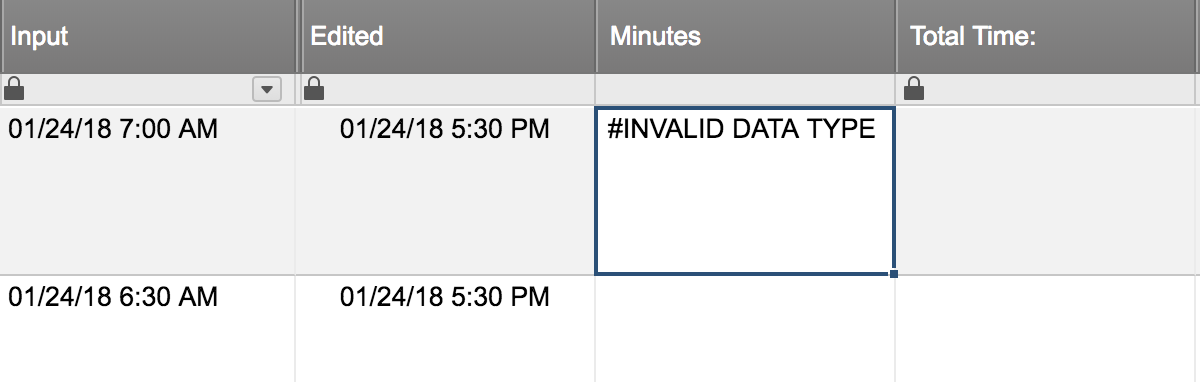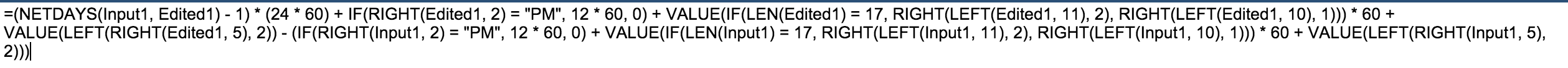# Time Formula - Drop Down Selection

Hello,

I've searched and it appears the time formula is an ongoing battle for enhancement requests. Looking for some help, I have two columns; 'Start Time:' and 'End Time'. There is a drop-down box on the web form and sheet that starts at 12:00 AM and ends at 11:55 PM, all increments of 5 minutes. For example, 12:00 AM, 12:05 AM, etc...

I've used the ginormous formula:

=IF([Start Time:]1 <> "", INT((((IF(LEFT([End Time:]1, FIND(":", [End Time:]1) - 1) = "12", IF(OR(FIND("a", [End Time:]1) > 0, FIND("p", [End Time:]1) > 0), 0, 12), VALUE(LEFT([End Time:]1, FIND(":", [End Time:]1) - 1))) + IF(FIND("p", [End Time:]1) > 0, 12)) * 60 + VALUE(MID([End Time:]1, FIND(":", [End Time:]1) + 1, 2))) - ((IF(LEFT([Start Time:]1, FIND(":", [Start Time:]1) - 1) = "12", IF(OR(FIND("a", [Start Time:]1) > 0, FIND("p", [Start Time:]1) > 0), 0, 12), VALUE(LEFT([Start Time:]1, FIND(":", [Start Time:]1) - 1))) + IF(FIND("p", [Start Time:]1) > 0, 12)) * 60 + VALUE(MID([Start Time:]1, FIND(":", [Start Time:]1) + 1, 2)))) / 60) + ":" + IF(((((IF(LEFT([End Time:]1, FIND(":", [End Time:]1) - 1) = "12", IF(OR(FIND("a", [End Time:]1) > 0, FIND("p", [End Time:]1) > 0), 0, 12), VALUE(LEFT([End Time:]1, FIND(":", [End Time:]1) - 1))) + IF(FIND("p", [End Time:]1) > 0, 12)) * 60 + VALUE(MID([End Time:]1, FIND(":", [End Time:]1) + 1, 2))) - ((IF(LEFT([Start Time:]1, FIND(":", [Start Time:]1) - 1) = "12", IF(OR(FIND("a", [Start Time:]1) > 0, FIND("p", [Start Time:]1) > 0), 0, 12), VALUE(LEFT([Start Time:]1, FIND(":", [Start Time:]1) - 1))) + IF(FIND("p", [Start Time:]1) > 0, 12)) * 60 + VALUE(MID([Start Time:]1, FIND(":", [Start Time:]1) + 1, 2)))) - INT((((IF(LEFT([End Time:]1, FIND(":", [End Time:]1) - 1) = "12", IF(OR(FIND("a", [End Time:]1) > 0, FIND("p", [End Time:]1) > 0), 0, 12), VALUE(LEFT([End Time:]1, FIND(":", [End Time:]1) - 1))) + IF(FIND("p", [End Time:]1) > 0, 12)) * 60 + VALUE(MID([End Time:]1, FIND(":", [End Time:]1) + 1, 2))) - ((IF(LEFT([Start Time:]1, FIND(":", [Start Time:]1) - 1) = "12", IF(OR(FIND("a", [Start Time:]1) > 0, FIND("p", [Start Time:]1) > 0), 0, 12), VALUE(LEFT([Start Time:]1, FIND(":", [Start Time:]1) - 1))) + IF(FIND("p", [Start Time:]1) > 0, 12)) * 60 + VALUE(MID([Start Time:]1, FIND(":", [Start Time:]1) + 1, 2)))) / 60) * 60) < 10, "0") + ((((IF(LEFT([End Time:]1, FIND(":", [End Time:]1) - 1) = "12", IF(OR(FIND("a", [End Time:]1) > 0, FIND("p", [End Time:]1) > 0), 0, 12), VALUE(LEFT([End Time:]1, FIND(":", [End Time:]1) - 1))) + IF(FIND("p", [End Time:]1) > 0, 12)) * 60 + VALUE(MID([End Time:]1, FIND(":", [End Time:]1) + 1, 2))) - ((IF(LEFT([Start Time:]1, FIND(":", [Start Time:]1) - 1) = "12", IF(OR(FIND("a", [Start Time:]1) > 0, FIND("p", [Start Time:]1) > 0), 0, 12), VALUE(LEFT([Start Time:]1, FIND(":", [Start Time:]1) - 1))) + IF(FIND("p", [Start Time:]1) > 0, 12)) * 60 + VALUE(MID([Start Time:]1, FIND(":", [Start Time:]1) + 1, 2)))) - INT((((IF(LEFT([End Time:]1, FIND(":", [End Time:]1) - 1) = "12", IF(OR(FIND("a", [End Time:]1) > 0, FIND("p", [End Time:]1) > 0), 0, 12), VALUE(LEFT([End Time:]1, FIND(":", [End Time:]1) - 1))) + IF(FIND("p", [End Time:]1) > 0, 12)) * 60 + VALUE(MID([End Time:]1, FIND(":", [End Time:]1) + 1, 2))) - ((IF(LEFT([Start Time:]1, FIND(":", [Start Time:]1) - 1) = "12", IF(OR(FIND("a", [Start Time:]1) > 0, FIND("p", [Start Time:]1) > 0), 0, 12), VALUE(LEFT([Start Time:]1, FIND(":", [Start Time:]1) - 1))) + IF(FIND("p", [Start Time:]1) > 0, 12)) * 60 + VALUE(MID([Start Time:]1, FIND(":", [Start Time:]1) + 1, 2)))) / 60) * 60), "")

This worked great UNTIL I changed it to AM / PM as my employees are struggling with military time. Any suggestions or have there been any improvements that I may have missed searching?

Secondly, is there a simple way to convert the increments when totaling the difference into decimal for the minutes?  Example: 7:05 PM - 10:00 PM converts to 2.92?

Regards,

Rockee

«1

• It is much easier to convert to minutes then output the hours.

Something like

IF(Right(Col1,2)="PM",12*60,0)+value(left(col1,2))*60+value(left(right(col1,5),2)

would be the minutes for one number. (assuming double digit hours. I didn't want to take the time to use len to correctly parse it, and you've already show you know how to do that.)

use the same formula to get the minutes for the other number and then subtract them. Then convert back into time.

• Realized I would only have to Parse the hours, so I went ahead and did it.

=IF(RIGHT([Start Time]1, 2) = "PM", 60 * 12, 0) + (VALUE(LEFT([Start Time]1, VALUE(LEN([Start Time]1) - 6))) * 60) + VALUE(LEFT(RIGHT([Start Time]1, 5), 2))

• Thanks for your reply Luke. I've tried your formula in a "Calculation" column and it gives an error: #UNPARSEABLE - I'll keep messing with it and see if I can get it going. You'd think something that is so simple on other platforms (Google Sheets and Excel) would be a no-brainer with Smartsheet.

• What is the format of the time you are putting in?

10:15 PM is what I used for that formula I just realized you have a ":" at the end of yours. That's probably the issue

• Give that a Try

=IF(RIGHT([End Time:]1, 2) = "PM", 60 * 12, 0) + (VALUE(LEFT([End Time:]1, VALUE(LEN([End Time:]1) - 6))) * 60) + VALUE(LEFT(RIGHT([End Time:]1, 5), 2)) - (IF(RIGHT([Start Time:]1, 2) = "PM", 60 * 12, 0) + (VALUE(LEFT([Start Time:]1, VALUE(LEN([Start Time:]1) - 6))) * 60) + VALUE(LEFT(RIGHT([Start Time:]1, 5), 2)))

• =ROUND(MinDiff1 / 60) + "H" + (MinDiff1 / 60 - ROUND(MinDiff1 / 60)) * 60 + "M"

This formula takes a minute output in column "MinDiff" row 1 and converts it to hours and minutes.

I would use a hidden helper column post the other formula to it, reference it, then hide it to get your number.

Lol got me interested. I had to build this once before, and had forgotten some of it.

Edited: Cleaned up the formula @1:53

• This formula seems to be off time wise when putting in minutes (7:25 AM - 9:00 PM).

=ROUND(MinDiff1 / 60) + "H" + (MinDiff1 / 60 - ROUND(MinDiff1 / 60)) * 60 + "M"

I ended up just taking the duration; =IF(RIGHT([End Time:]1, 2) = "PM", 60 * 12, 0) + (VALUE(LEFT([End Time:]1, VALUE(LEN([End Time:]1) - 6))) * 60) + VALUE(LEFT(RIGHT([End Time:]1, 5), 2)) - (IF(RIGHT([Start Time:]1, 2) = "PM", 60 * 12, 0) + (VALUE(LEFT([Start Time:]1, VALUE(LEN([Start Time:]1) - 6))) * 60) + VALUE(LEFT(RIGHT([Start Time:]1, 5), 2))) and dividing by 60 which also gives me the minutes in decimals. My last issue is to figure out what to do if they end a job a midnight as none of the formulas like that and turn it into negative time.

Thanks for all of your help.

• I forgot to subtract .5 from the rounds to make sure it rounds down. That should correct the issue i would think.

I Went back to an old sheet and reviewed it and edited it. It is a little deeper than this one as it adds days as well, which could be an option for your midnight problem. (honestly just rename your columns copy paste the formulas, then name your columns back) this way you could track days as well as time. There is a little glitch I haven't figured out where is there is less than a minute between the start and stop it goes to -23H but I don't think that's a huge deal. I just used conditional formatting to make it blank if that happens. There's not really any point in tracking anything less than 1 min in smartsheet.

Click the check boxes then save to refresh the row. i've got a personal copy of the sheet so don't worry about messing it up.

https://app.smartsheet.com/b/publish?EQBCT=dcf71ecb7f184467ab8f5debb68a04da

• Awesome Luke!

That gave me some ideas! I did some error checking and then JOINed the Date and Time, changed the column names to match yours and I'm getting an error - #INVALID DATA TYPE. See anything I may have missed (attached screen shot).• can you post the equation in a response? Also, check the column properties and make sure it is text not date.

• Had one column (Edited) that was set to Date, although that didn't change the output in the "Minutes" column.

=(NETDAYS(Input1, Edited1) - 1) * (24 * 60) + IF(RIGHT(Edited1, 2) = "PM", 12 * 60, 0) + VALUE(IF(LEN(Edited1) = 17, RIGHT(LEFT(Edited1, 11), 2), RIGHT(LEFT(Edited1, 10), 1))) * 60 + VALUE(LEFT(RIGHT(Edited1, 5), 2)) - (IF(RIGHT(Input1, 2) = "PM", 12 * 60, 0) + VALUE(IF(LEN(Input1) = 17, RIGHT(LEFT(Input1, 11), 2), RIGHT(LEFT(Input1, 10), 1))) * 60 + VALUE(LEFT(RIGHT(Input1, 5), 2)))